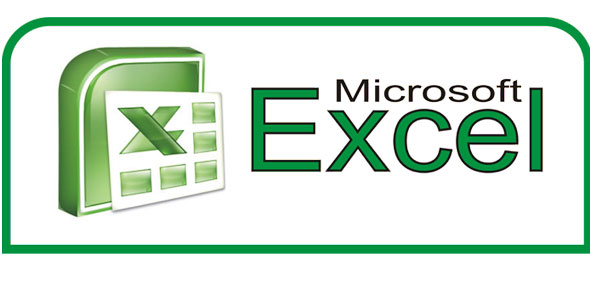# Microsoft Excel 2003 Exam 2

25 Questions | Total Attempts: 199SettingsINSTRUCTIONS: 1. Read all questions CAREFULLY! 2. Attempt ALL questions. 3. Total time allotted is 1. 5 hours.

• 1.
To create macro buttons, you will display the __________ toolbar.
• A.

Standard

• B.

Formatting

• C.

Drawing

• D.

Forms

• 2.
When creating a two-variable data table, you must identify the _____ input Cell
• A.

Variable

• B.

Column Only

• C.

• D.

Row and Column

• 3.
The message "#DIV/0!" means that
• A.

You instructed Excel to divide the number in the cell by zero

• B.

The resulting value is too long for the field

• C.

The resulting value is not a whole number

• D.

You have not designated a format for the resulting answer

• 4.
In a non-interactive web page the user can __________.
• A.

Scroll through the contents and make changes

• B.

Scroll through the contents and only make comment

• C.

Scroll through the contents but cannot make any changes

• D.

Not switch between worksheets

• 5.
You can assign range names of up to __________ characters.
• A.

12

• B.

24

• C.

255

• D.

526

• 6.
The first argument in the VLOOKUP function is the __________ value.
• A.

Lookup

• B.

Range

• C.

Array

• D.

Index

• 7.
The first component of CVP analysis is ___________
• A.

Cost

• B.

Revenue

• C.

Pricing

• D.

All Of the Above

• 8.
To quickly go to the cell referenced by name, you can select the name from the  __________.
• A.

Text Box

• B.

Formula Box

• C.

Name Box

• D.

Insert Box

• 9.
In the 3-D cell reference, 'E:\Business\[Sales.xls]Summary Info'!B5:E5, theworkbook name is
• A.

• B.

[Sales.xls]

• C.

Summary Info

• D.

B5:E5

• 10.
Raw material is a(n) __________ expense because the expenses increase with higher quantities produced.
• A.

Fixed

• B.

Multifaceted

• C.

Variable

• D.

Mixed

• 11.
Which of the following Solver reports is used primarily in science and engineering environments?
• A.

• B.

Scenario Report

• C.

Lookup Report

• D.

Limits of sensitivity

• 12.
By default, Excel uses __________ references while recording macros.
• A.

Mixed

• B.

Relative

• C.

Absolute

• D.

Dynamic

• 13.
To select consecutive worksheets, press and hold down the __________ key, and then click the last sheet tab you want to include.
• A.

Shift

• B.

Ctrl

• C.

Alt

• D.

F1

• 14.
You enter 3-D cell references by
• A.

Using double quotations in the reference

• B.

Entering the cell ranges followed by the sheet name

• C.

Using the Reference Wizard

• D.

Selecting the appropriate cells with the mouse as you enter the formula or by typing the reference

• 15.
Whenever you start Excel and see the blank workbook called __________, you are using a workbook that is based on a template known as the default template.
• A.

Sheet 1

• B.

Worksheet 1

• C.

Book 1

• D.

Workbook 1

• 16.
The __________ cell is the cell whose input values are placed in the first column of a data table.
• A.

Row input

• B.

Primary data

• C.

Column Input

• D.

Value

• 17.
One way to ensure that correct data is entered into a cell or range is to use the Excel __________ feature.
• A.

Data Check

• B.

Data Overview

• C.

Data Validation

• D.

Data Type

• 18.
The Set Target Cell box in the Solver parameters dialog box must contain the cell reference for the __________ cell.
• A.

Application

• B.

Target

• C.

Worksheet

• D.

Basis

• 19.
To create a template, you simply save an Excel workbook as a template file using the __________ command.
• A.

Save As

• B.

Template

• C.

Save

• D.

Store As

• 20.
Goal Seek can change the contents of __________ cell(s) to find a solution.
• A.

One

• B.

Two

• C.

Three

• D.

Four or more

• 21.
You must place an order for refrigerators with the restrictions that the cost of the order cannot exceed \$18,000 and that the available space is limited to a maximum of 1,000 cubic feet; which of the following are goal-seeking questions related to this problem?
• A.

How much does 1 refrigerator cost?

• B.

How many refrigerators can fit into 5 square yard

• C.

How many cubic feet does \$18,000 worth of refrigerators hold

• D.

How many refrigerators can I order with \$18,000 and fill up to a 1,000 cubic space?

• 22.
To retain the Solver solutions, select the __________ option button in the Solver Results dialog box.
• A.

• B.

Keep Solver Solution

• C.

Save Solver

• D.

Solver Save

• 23.
To deal with problems with ________ input cell(s), you have to create scenarios.
• A.

One

• B.

Two

• C.

More than two

• D.

All of the above

• 24.
To open the Solver parameters dialog box, click Tools, and then click
• A.

• B.

Templates

• C.

Solver

• D.

More Tools

• 25.
How can you tell if a worksheet is being shared?
• A.

The word Exported is displayed in the Excel title bar

• B.

The word Shared is displayed in the Excel title bar

• C.

You can not tell if it is being shared

• D.

The word Shared is displayed in the Word title bar

Related TopicsBack to top